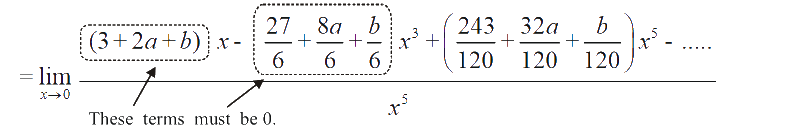# Limits Continuity Differentiability And Differentiation Set-3

Go back to  'SOLVED EXAMPLES'

Learn from the best math teachers and top your exams

• Live one on one classroom and doubt clearing
• Practice worksheets in and after class for conceptual clarity
• Personalized curriculum to keep up with school

Example – 9

If   f(x) = \left\{ \begin{align}&\frac{{\sin 3x + a\sin 2x + b\sin x}}{{{x^5}}}, & x \ne 0\\&\qquad \qquad\quad c & , \quad x = 0 \end{align} \right\} is continuous at x = 0, find a, b and c.

Solution:    For continuity, we require:

\begin{align}&\mathop {\lim }\limits_{x \to 0} f(x) = c \quad \left\{ \begin{array}{l}{\rm{LHL\,and\,RHL\,will\,be\,the\,same\,so\,we }}\\{\rm{do\,not\,need\,to\,evaluate\,them\, separately}}\end{array} \right\}\\\\&\mathop {\lim }\limits_{x \to 0} f(x) = \mathop {\lim }\limits_{x \to 0} \left\{ {\frac{{\sin 3x + a\sin 2x + b\sin x}}{{{x^5}}}} \right\}\end{align}

\begin{align}& = \mathop {\lim }\limits_{x \to 0} \frac{{\left\{ {3x - \frac{{{{(3x)}^3}}}{{3!}} + \frac{{{{(3x)}^5}}}{{5!}} - ....} \right\} + a\left\{ {2x - \frac{{{{(2x)}^3}}}{{3!}} + \frac{{{{(2x)}^5}}}{{5!}} - .....} \right\} + b\left\{ {x - \frac{{{x^3}}}{{3!}} + \frac{{{x^5}}}{{5!}} - ...} \right\}}}{{{x^5}}}\\\end{align}As in Section -1, example-3, note that

$3{\rm{\ }} + {\rm{ }}2a + b = {\rm{ }}0\qquad\qquad\qquad...{\rm{ }}\left( i \right)$

$27{\rm{ }} + {\rm{ }}8a + b = {\rm{ }}0{\rm{ }}\qquad\qquad\qquad...{\rm{ }}\left( {ii} \right)$

\left\{ \begin{align}&{\rm{If\,this\,does\,not\,hold,}}\\ & \mathop {\lim }\limits_{x \to 0} \frac{{(3 + 2a + b)x}}{{{x^5}}}{\rm{ \;and\;}}\mathop {\lim }\limits_{x \to 0} \frac{{(27 + 8a + b){x^3}}}{{{x^5}}}\\&{\rm{will\,become\,infinite}}\end{align} \right\}

Solving (i) and (ii), we get

$$a = {\rm{ }}-{\rm{ }}4{\rm{ }}\qquad\qquad{\rm{ }}b = {\rm{ }}5$$

The limit now reduces to

\begin{align}&\mathop {\lim }\limits_{x \to 0} \frac{{\left( {\frac{{243}}{{120}} + \frac{{32a}}{{120}} + \frac{b}{{120}}} \right){x^5} + ....\left( {{\rm{higher}}\,{\rm{order}}\,{\rm{terms}}} \right)}}{{{x^5}}}\\& = \frac{{243 + 32a + b}}{{120}}\\& = {\rm{ }}1\end{align}

Hence,

c = 1

Example - 10

Let   $$f:\mathbb{R} \to \mathbb{R}$$  be a function defined by $$f\left( {x + y} \right) = f\left( x \right)f\left( y \right)$$  for all   $$x,y \in \mathbb{R}.$$ If     $$f\left( x \right)$$ is continuous at    x = 0, show that $$f\left( x \right)$$  is continuous for all $$x \in \mathbb{R}.$$

Solution:    Since   $$f\left( x \right)$$  is continuous at   $$x = 0,$$

$$\mathop {\lim }\limits_{h \to 0} f\left( { - h} \right) = \mathop {\lim }\limits_{h \to 0} f\left( h \right) = f\left( 0 \right)$$               ... (i)

To evaluate  $$f\left( 0 \right),$$  we substitute $$x = y = 0$$  in the given functional relation to get.

\begin{align}&\quad\quad{\rm{ }}f\left( 0 \right) = f{\left( 0 \right)^2}\\ &\Rightarrow f\left( 0 \right) = 0\,\,{\rm{or}}\,\,\,f\left( 0 \right) = 1\end{align}

(i)    If $$f\left( 0 \right) = 0,$$  then $$f\left( x \right) = f\left( {x + 0} \right) = f\left( x \right) \cdot f\left( 0 \right) = 0$$   i.e

$$f\left( x \right) = 0$$    for all values of x so that $$f\left( x \right)$$   is continuous everywhere.

(ii)      We now assume $$f\left( 0 \right) = 1.$$

Now,

LHL at any $$x = \mathop {\lim }\limits_{h \to 0} f\left( {x - h} \right)$$

\begin{align}& = \mathop {\lim }\limits_{h \to 0} f\left( x \right) \cdot f\left( { - h} \right)\\& = f\left( x \right)\mathop {\lim }\limits_{h \to 0} f\left( { - h} \right)\\ &= f\left( x \right) \cdot f\left( 0 \right){\rm{ }}\left( {From{\rm{ }}\left( i \right)} \right)\\ &= f\left( x \right)\end{align}

Similarly,

RHL at any $$x = \mathop {\lim }\limits_{h \to 0} f\left( {x + h} \right)$$

\begin{align}& = \mathop {\lim }\limits_{h \to 0} f\left( x \right)f\left( h \right) \\&= f\left( x \right)\end{align}

Hence, $$f\left( x \right)$$   is continuous for all     $$x \in \mathbb{R}$$

Learn from the best math teachers and top your exams

• Live one on one classroom and doubt clearing
• Practice worksheets in and after class for conceptual clarity
• Personalized curriculum to keep up with school

0